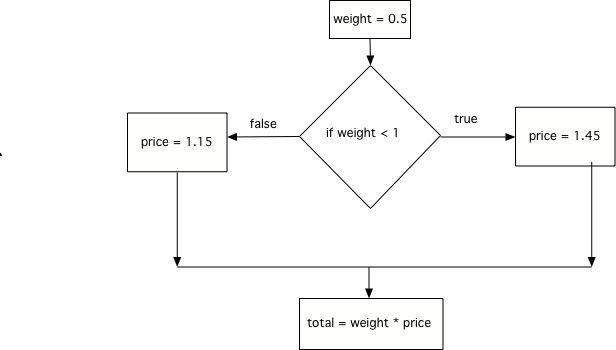# 12.7. Using if and else¶

Most professional programmers would write the following code:

Like this:

An `else` is an additional optional phrase on an `if` statement. IF AND ONLY IF the test in the `if` is false does the block of statements after the `else` get executed. Using an `if` with an `else` makes sure that either the `if` block is executed or the `else` block is executed, but never both.Figure 4: Flow of execution for both an if and else

Mixed up programs

The following program should print out “x is even” if the remainder of x divided by 2 is 0 and “x is odd” otherwise, but the code is mixed up. The `%` symbol gives the remainder after the first number is divided by the second number. Drag the blocks from the left and place them in the correct order on the right. Be sure to also indent correctly! Click on <i>Check Me</i> to see if you are right. You will be told if any of the lines are in the wrong order or have the wrong indention.</p>

```        x = 92
if x % 2 == 0:
print("x is even")
else:
print("x is odd")
```

It is easy to write an `if` when you want exactly one block to execute, but you can accidentally create a “hole” – a condition where neither block executes. That’s what happened in the example below when the weight is equal to 1 pound.

Fix the example above such that the cost of frozen yogurt is 0 if you pour exactly 1 lb. in your cup.